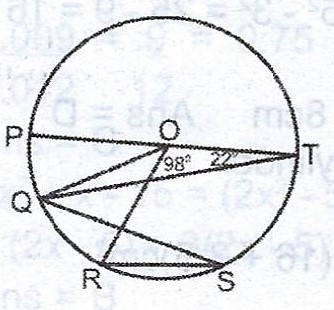# (a) (i) Prove that the angle which an arc of a circle subtends at the centre is twice that which it subtends at any point on the remaining part of the circu...

Question 1

(a) (i) Prove that the angle which an arc of a circle subtends at the centre is twice that which it subtends at any point on the remaining part of the circumference.(ii) In the diagram above, O is the centre of the circle and PT is a diameter. If < PTQ = 22° and < TOR = 98°, calculate < QRS.

(b) ABCD is a cyclic quadrilateral and the diagonals AC and BD intersect at H. If < DAC = 41° and < AHB = 70°, calculate < ABC.